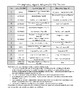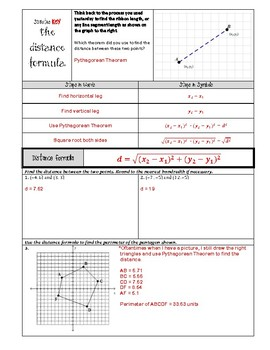# High School Math 1: Congruence, Algebra, and GeometrySubject
Resource Type
Format
PDF (6 MB|81 pages)
Standards
\$14.00
• Product Description
• Standards
Task-Based Learning immediately engages students in problem-solving and mathematical thinking. No song and dance necessary - your students will be intrinsically motivated to learn more. This unit thoroughly covers the concept of congruence and verifying congruence on the coordinate plane using the distance formula. This unit also covers triangle congruence and polygon symmetries.

This unit is designed for a Secondary Mathematics One Course (usually 9th graders) and includes:
- Tasks to develop mathematical thinking
- Notes to solidify mathematical concepts
- Activities to practice mathematical concepts in class
- Homework to practice mathematical concepts individually
- A mid-unit and end-of-unit assessment
- Full keys and teacher notes

All of this is immediately ready to print and copy for student use. Give this teaching method a try and I promise you will see growth in your students.
to see state-specific standards (only available in the US).
Use coordinates to compute perimeters of polygons and areas of triangles and rectangles, e.g., using the distance formula.
Prove the slope criteria for parallel and perpendicular lines and use them to solve geometric problems (e.g., find the equation of a line parallel or perpendicular to a given line that passes through a given point).
Use coordinates to prove simple geometric theorems algebraically. For example, prove or disprove that a figure defined by four given points in the coordinate plane is a rectangle; prove or disprove that the point (1, √3) lies on the circle centered at the origin and containing the point (0, 2).
Explain how the criteria for triangle congruence (ASA, SAS, and SSS) follow from the definition of congruence in terms of rigid motions.
Use the definition of congruence in terms of rigid motions to show that two triangles are congruent if and only if corresponding pairs of sides and corresponding pairs of angles are congruent.
Total Pages
81 pages
Included with rubric
Teaching Duration
3 Weeks
Report this Resource to TpT
Reported resources will be reviewed by our team. Report this resource to let us know if this resource violates TpT’s content guidelines.
• Ratings & Reviews
• Q & A

Teachers Pay Teachers is an online marketplace where teachers buy and sell original educational materials.